# MCQs on Electric Circuits

##### Page 7 of 63. Go to page 1 2 3 4 5 6 7 8 9 10 11 12 13 14 15 16 17 18 19 20 21 22 23 24 25 26 27 28 29 30 31 32 33 34 35 36 37 38 39 40 41 42 43 44 45 46 47 48 49 50 51 52 53 54 55 56 57 58 59 60 61 62 63
01․ At which of the following frequency voltage across capacitor has maximum value for series circuit ?
Equal to resonance frequency
Less than resonance frequency
Greater than resonance frequency
Zero frequency

At resonance frequency voltage across capacitor and inductor are same, but this is not the maximum value. The maximum value across capacitor will occur below resonance frequency. Because voltage across the capacitor is inversely proportional to its frequency.

02․ To obtain high efficiency, network is designed with which of the following?
High Q factor
Low Q factor
Unity Q factor
Zero Q factor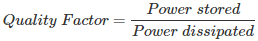Since Q factor is inversely proportional to power loss and directly proportional to efficiency.

03․ To obtain wide bandwidth, network is designed with which of the following?
High Q factor
Low Q factor
Unity Q factor
Zero Q factor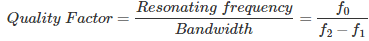Since bandwidth is inversely proportional to Q factor, to obtained wide bandwidth quality factor must be low.

04․ In series RLC circuit which of the following represents the Q factor ?
XC/R
VR/V
XL/R
All of the above

These all are the different forms of representing Q factor quality factor in a RLC circuit.

05․ In series RLC circuit what is the power factor just bellow the resonance frequency?
Lagging
Unity
Zero

At series resonance, capacitive reactance is same as inductive reactance in value. As the capacitive reactance is inversely proportional to frequency hence below the resonance frequency in series RLC circuit, the capacitive reactance is more than inductive reactance. Therefore power factor is leading.

06․ In series RLC circuit what is the power factor just above the resonance frequency?
Lagging
Unity
Zero

At series resonance, capacitive reactance is same as inductive reactance in value. As the inductive reactance is directly proportional to frequency and capacitive reactance is inversely proportional to frequency, above the resonance frequency in series RLC circuit, the inductive reactance is more than capacitive reactance. Therefore power factor is lagging.

07․ Which of the following represents the resonance frequency (ω0) with respect to lower cutoff frequency (ω1) and higher cutoff frequency (ω2)
ω1 + ω2
ω1 - ω2
ω1 / ω2
√(ω1² + ω2²)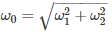08․ In a series resonance circuit if bandwidth is 1 MHz and inductance is 1 mH, then what is the resistance value?
1 KΩ
1 MΩ
1 Ω
100 Ω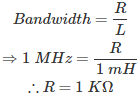09․ In RLC series circuit if the Q factor is 1, then the damping of the system is
Critical damping
Over damping
Under damping
Undamping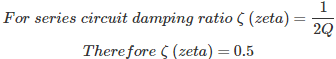10․ In RLC series circuit, at resonance condition the value of current is
Maximum
Minimum
Zero
None of the above

In series RLC network under resonance condition the value of inductive reactance is equal to the capacitive reactance and they will cancel each other. Therefore impedance become equals to resistance and is minimum under resonance condition and hence current is maximum.

<<<56789>>>